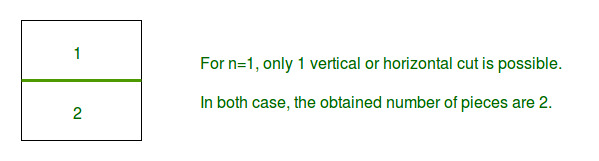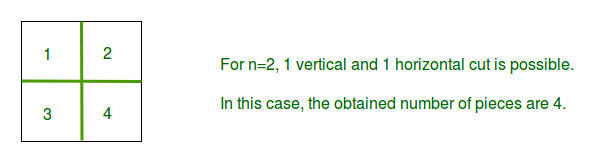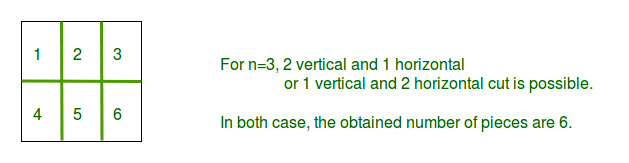# Maximum number of pieces in N cuts

Given a square piece and a total number of cuts available n, Find out the maximum number of rectangular or square pieces of equal size that can be obtained with n cuts. The allowed cuts are horizontal and vertical cut.
Note: Stacking and folding is not allowed.
Examples

```Input : n = 1
Output : 2
Explanation : ``````Input : n = 2
Output : 4
Explanation : ``````Input : n = 3
Output : 6
Explanation : ```` `

Given is n which is the number of allowed cuts. As it is required to maximize number of pieces after n cuts, So number of horizontal cuts will be equal to number of vertical cuts. This can be prove using differentiation. So number of horizontal cut will be n/2. and vertical cuts will be n-n/2.
So number of pieces = (horizontal cut + 1) * (vertical cut + 1).
Program:

## C++

 `// C++ program to find maximum no of pieces` `// by given number of cuts` `#include ` `using` `namespace` `std;`   `// Function for finding maximum pieces` `// with n cuts.` `int` `findMaximumPieces(``int` `n)` `{` `    ``// to maximize number of pieces` `    ``// x is the horizontal cuts` `    ``int` `x = n / 2;`   `    ``// Now (x) is the horizontal cuts` `    ``// and (n-x) is vertical cuts, then` `    ``// maximum number of pieces = (x+1)*(n-x+1)` `    ``return` `((x + 1) * (n - x + 1));` `}`   `// Driver code` `int` `main()` `{`   `    ``// Taking the maximum number of cuts allowed as 3` `    ``int` `n = 3;`   `    ``// Finding and printing the max number of pieces` `    ``cout << ``"Max number of pieces for n = "` `<< n` `         ``<< ``" is "` `<< findMaximumPieces(3);`   `    ``return` `0;` `}`

## Java

 `// Java program to find maximum ` `// no of pieces by given number` `// of cuts ` `import` `java.util.*;`   `class` `GFG` `{` `// Function for finding maximum` `// pieces with n cuts. ` `public` `static` `int` `findMaximumPieces(``int` `n) ` `{ ` `    ``// to maximize number of pieces ` `    ``// x is the horizontal cuts ` `    ``int` `x = n / ``2``; `   `    ``// Now (x) is the horizontal cuts ` `    ``// and (n-x) is vertical cuts, then ` `    ``// maximum number of pieces = (x+1)*(n-x+1) ` `    ``return` `((x + ``1``) * (n - x + ``1``)); ` `} `   `// Driver code` `public` `static` `void` `main (String[] args) ` `{` `    ``// Taking the maximum number ` `    ``// of cuts allowed as 3 ` `    ``int` `n = ``3``; ` `    `  `    ``// Finding and printing the ` `    ``// max number of pieces ` `    ``System.out.print(``"Max number of pieces for n = "` `+` `                   ``n + ``" is "` `+ findMaximumPieces(``3``)); ` `        `  `}` `}`   `// This code is contributed by Kirti_Mangal`

## Python 3

 `# Python 3 program to find maximum no of pieces` `# by given number of cuts` ` `  `# Function for finding maximum pieces` `# with n cuts.` `def` `findMaximumPieces(n):`   `    ``# to maximize number of pieces` `    ``# x is the horizontal cuts` `    ``x ``=` `n ``/``/` `2` ` `  `    ``# Now (x) is the horizontal cuts` `    ``# and (n-x) is vertical cuts, then` `    ``# maximum number of pieces = (x+1)*(n-x+1)` `    ``return` `((x ``+` `1``) ``*` `(n ``-` `x ``+` `1``))` ` `  `# Driver code` `if` `__name__ ``=``=` `"__main__"``:` ` `  `    ``#Taking the maximum number of cuts allowed as 3` `    ``n ``=` `3` ` `  `    ``# Finding and printing the max number of pieces` `    ``print``(``"Max number of pieces for n = "` `+``str``( n)` `         ``+``" is "` `+` `str``(findMaximumPieces(``3``)))`   `# This code is contributed by ChitraNayal`

## C#

 `// C# program to find maximum ` `// no of pieces by given number` `// of cuts ` `using` `System;`   `class` `GFG` `{`   `// Function for finding maximum` `// pieces with n cuts. ` `public` `static` `int` `findMaximumPieces(``int` `n) ` `{ ` `    ``// to maximize number of pieces ` `    ``// x is the horizontal cuts ` `    ``int` `x = n / 2; `   `    ``// Now (x) is the horizontal  ` `    ``// cuts and (n-x) is vertical ` `    ``// cuts, then maximum number ` `    ``// of pieces = (x+1)*(n-x+1) ` `    ``return` `((x + 1) * (n - x + 1)); ` `} `   `// Driver code` `static` `public` `void` `Main ()` `{` `    ``// Taking the maximum number ` `    ``// of cuts allowed as 3 ` `    ``int` `n = 3; ` `    `  `    ``// Finding and printing the ` `    ``// max number of pieces ` `    ``Console.Write(``"Max number of pieces for n = "` `+` `                ``n + ``" is "` `+ findMaximumPieces(3)); ` `}` `}`   `// This code is contributed by Mahadev`

## PHP

 ``

## Javascript

 ``

Output:

`Max number of pieces for n = 3 is 6`

Time Complexity: O(1)
Auxiliary Space: O(1)

Feeling lost in the world of random DSA topics, wasting time without progress? It's time for a change! Join our DSA course, where we'll guide you on an exciting journey to master DSA efficiently and on schedule.
Ready to dive in? Explore our Free Demo Content and join our DSA course, trusted by over 100,000 geeks!